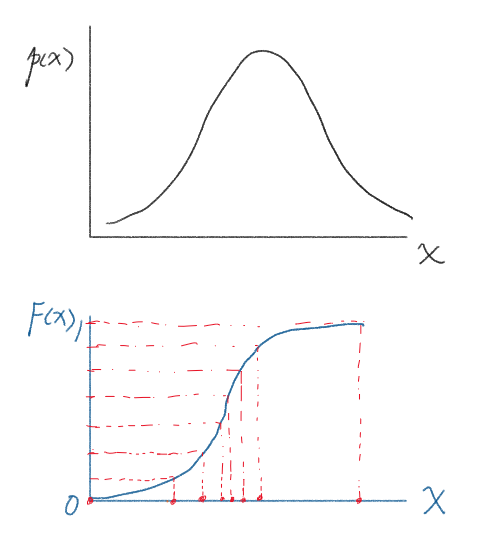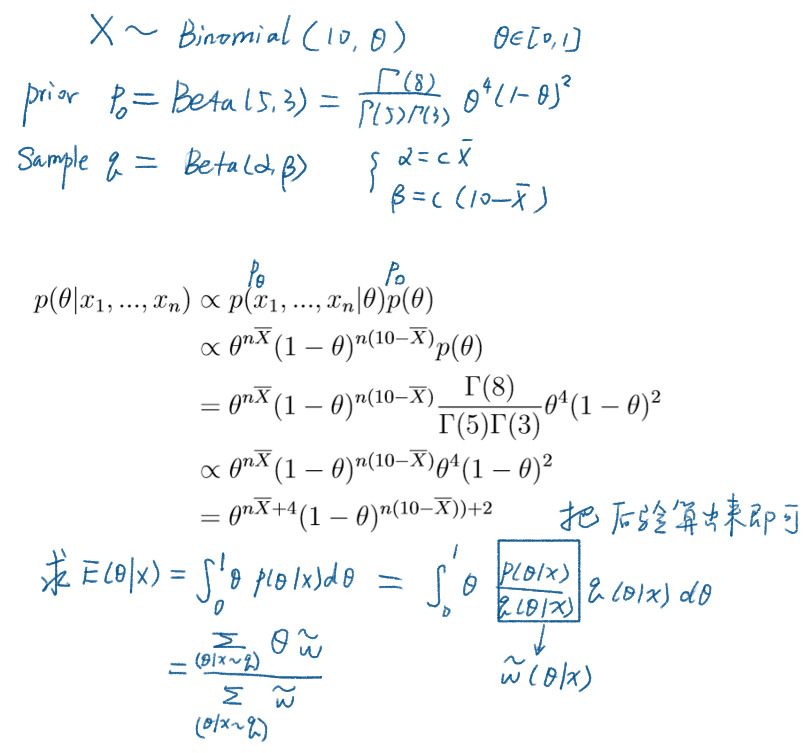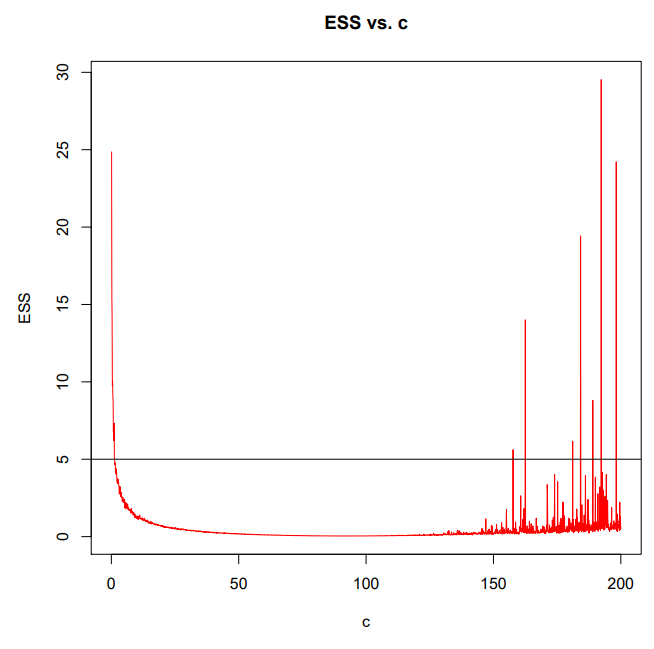## 采样## 贝叶斯推理

C 同样可以采样得到：

$p(X|\theta)$ 是模型假设；$p(\theta)$ 和 $q(\theta)$ 是形式相同的两个分布。$q$ 可能会根据输入数据的特性来调整。## q 的选取

$q$ 在 $p$ 高密度的地方也要尽量高密度，这样才能真实还原数据的性质。## Reference

Importance Sampling